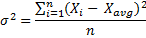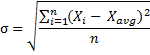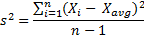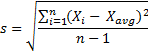# Population vs. Sample Variance and Standard Deviation

You can easily calculate population or sample variance and standard deviation, as well as skewness, kurtosis, and other measures, using the Descriptive Statistics Excel Calculator.

## Variance and Standard Deviation Definition and Calculation

Variance and standard deviation are widely used measures of dispersion of data or, in finance and investing, measures of volatility of asset prices.

Variance is defined and calculated as the average squared deviation from the mean. Standard deviation is calculated as the square root of variance or in full definition, standard deviation is the square root of the average squared deviation from the mean.

These definitions may sound confusing when encountered for the first time. For detailed explanation how to calculate both measures see Calculating Variance and Standard Deviation in 4 Easy Steps.

## Describing vs. Forecasting in Statistics

In general statistics performs two main tasks. Its goal is either to describe something that has already happened or already exists (descriptive statistics), or to estimate something that has not happened yet or is not fully known (inferential statistics).

Descriptive statistics deals with the problem how to effectively look at data we already have. Inferential statistics (the estimating and forecasting part of statistics) deals with the problem of not having all the data.

Of these two broad areas of statistics, inferential statistics is the one that is much more interesting and much more frequently used in finance and investing. After all, as investors or speculators, we often have to face the problem that we want to know what we don't know (for example, whether stock XYZ will go up or down tomorrow and by how much).

## Population vs. Sample

The primary task of inferential statistics (or estimating or forecasting) is making an opinion about something by using only an incomplete sample of data.

In statistics it is very important to distinguish between population and sample. A population is defined as all members (e.g. occurrences, prices, annual returns) of a specified group. Population is the whole group.

A sample is a part of a population that is used to describe the characteristics (e.g. mean or standard deviation) of the whole population. The size of a sample can be less than 1%, or 10%, or 60% of the population, but it is never the whole population.

## Population vs. Sample Variance and Standard Deviation

When calculating variance and standard deviation, it is important to know whether we are calculating them for the whole population using all the data, or we are calculation them using only a sample of data. In the first case we call them population variance and population standard deviation. In the second case we call them sample variance and sample standard deviation.

## Example 1: Population Variance and Standard Deviation

Question: What is the standard deviation of last year's returns of the 12 funds I have invested in?

There is no estimating or forecasting in this task. I am only interested in the 12 funds I have invested in and I don't care about the thousands of other funds which exist in the world. My population is only these 12 funds. I have all the data available, as it is very easy to find these 12 funds' performance data.

I take the performance of each of the 12 funds in the last year, calculate the mean, then the deviations from the mean, square the deviations, sum the squared deviations up, divide by 12 (the number of funds), and get the variance. Then the square root of variance is the standard deviation. In this case, because I have the data for the whole population available, I call them population variance and population standard deviation.

## Example 2: Sample Variance and Standard Deviation

Question: What is the standard deviation of last year's returns of equity funds in the world?

Compared to calculating standard deviation of concretely specified 12 funds, I now want to know the standard deviation of returns of all equity funds in the world. My population is now much larger than in the previous example. There are thousands of equity funds in the world. Some of them probably aren't on the Bloomberg, don't have a website, and don't publish their performance. In short, I have no chance that I could get the data for all the funds. And even if I could, it would take a long time and cost a lot of money to get all the data.

Contrary to the previous example, I now don't have all the data available and I will have to estimate the population's standard deviation from a sample.

## Estimating Population Standard Deviation from a Sample

So how will I do it? I will try to collect the data for some of the equity funds – these funds will be my sample. It is not necessary (and probably not possible) to collect the data for all the funds in the world (the population). I must only make sure that my sample is large enough. While having the data for 5 funds would probably be insufficient to estimate standard deviation for the whole population, 100 funds' data can be enough and still very realistic to get.

Taking the data for these 100 funds I calculate the variance and standard deviation in the same way as in example 1 with my 12 funds.

## The Difference in Calculation: Population vs. Sample Variance

There is only one little difference in the calculation of variance and it is at the very end of it. For both population and sample variance, I calculate the mean, then the deviations from the mean, and then I square all the deviations. I sum all the squared deviations up. So far it was the same for both population and sample variance.

When I calculate population variance, I then divide the sum of squared deviations from the mean by the number of items in the population (in example 1 I was dividing by 12).

When I calculate sample variance, I divide it by the number of items in the sample less one. In our example 2, I divide by 99 (100 less 1).

As a result, the calculated sample variance (and therefore also the standard deviation) will be slightly higher than if we would have used the population variance formula. The purpose of this little difference it to get a better and unbiased estimate of the population‘s variance (by dividing by the sample size lowered by one, we compensate for the fact that we are working only with a sample rather than with the whole population).

In the guide to calculating variance and standard deviation we were calculating population variance and standard deviation. For sample variance and standard deviation, the only difference is in step 4, where we now divide by the number of items less one.

## Formulas

### Population Variance### Population Standard Deviation### Sample Variance### Sample Standard Deviation## Calculating Variance and Standard Deviation in Excel

In Excel, variance and standard deviation can be easily calculated using the built-in functions: VAR.P, VAR.S, STDEV.P, and STDEV.S (of course you can also calculate them directly using the formulas above if you like). You can see how the calculation works in practice (as well as the calculation of skewness, kurtosis, and other measures) in the Descriptive Statistics Excel Calculator.

By remaining on this website or using its content, you confirm that you have read and agree with the Terms of Use Agreement.

We are not liable for any damages resulting from using this website. Any information may be inaccurate or incomplete. See full Limitation of Liability.

Content may include affiliate links, which means we may earn commission if you buy on the linked website. See full Affiliate and Referral Disclosure.

We use cookies and similar technology to improve user experience and analyze traffic. See full Cookie Policy.